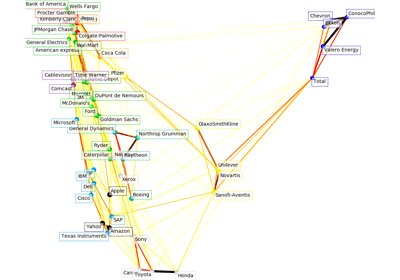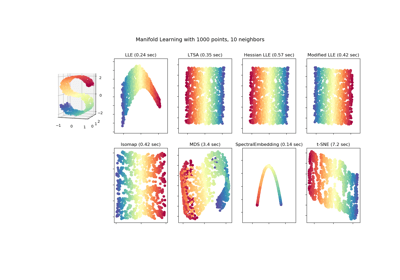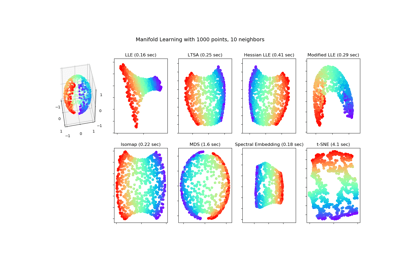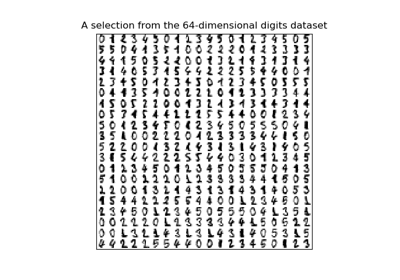/scikit-learn

# sklearn.manifold.LocallyLinearEmbedding

`class sklearn.manifold.LocallyLinearEmbedding(n_neighbors=5, n_components=2, reg=0.001, eigen_solver=’auto’, tol=1e-06, max_iter=100, method=’standard’, hessian_tol=0.0001, modified_tol=1e-12, neighbors_algorithm=’auto’, random_state=None, n_jobs=None)` [source]

Locally Linear Embedding

Read more in the User Guide.

Parameters: `n_neighbors : integer` number of neighbors to consider for each point. `n_components : integer` number of coordinates for the manifold `reg : float` regularization constant, multiplies the trace of the local covariance matrix of the distances. `eigen_solver : string, {‘auto’, ‘arpack’, ‘dense’}` auto : algorithm will attempt to choose the best method for input data `arpack : use arnoldi iteration in shift-invert mode.` For this method, M may be a dense matrix, sparse matrix, or general linear operator. Warning: ARPACK can be unstable for some problems. It is best to try several random seeds in order to check results. `dense : use standard dense matrix operations for the eigenvalue` decomposition. For this method, M must be an array or matrix type. This method should be avoided for large problems. `tol : float, optional` Tolerance for ‘arpack’ method Not used if eigen_solver==’dense’. `max_iter : integer` maximum number of iterations for the arpack solver. Not used if eigen_solver==’dense’. `method : string (‘standard’, ‘hessian’, ‘modified’ or ‘ltsa’)` `standard : use the standard locally linear embedding algorithm. see` reference  `hessian : use the Hessian eigenmap method. This method requires` `n_neighbors > n_components * (1 + (n_components + 1) / 2` see reference  `modified : use the modified locally linear embedding algorithm.` see reference  `ltsa : use local tangent space alignment algorithm` see reference  `hessian_tol : float, optional` Tolerance for Hessian eigenmapping method. Only used if `method == 'hessian'` `modified_tol : float, optional` Tolerance for modified LLE method. Only used if `method == 'modified'` `neighbors_algorithm : string [‘auto’|’brute’|’kd_tree’|’ball_tree’]` algorithm to use for nearest neighbors search, passed to neighbors.NearestNeighbors instance `random_state : int, RandomState instance or None, optional (default=None)` If int, random_state is the seed used by the random number generator; If RandomState instance, random_state is the random number generator; If None, the random number generator is the RandomState instance used by `np.random`. Used when `eigen_solver` == ‘arpack’. `n_jobs : int or None, optional (default=None)` The number of parallel jobs to run. `None` means 1 unless in a `joblib.parallel_backend` context. `-1` means using all processors. See Glossary for more details. `embedding_ : array-like, shape [n_samples, n_components]` Stores the embedding vectors `reconstruction_error_ : float` Reconstruction error associated with `embedding_` `nbrs_ : NearestNeighbors object` Stores nearest neighbors instance, including BallTree or KDtree if applicable.

#### References

  `Roweis, S. & Saul, L. Nonlinear dimensionality reduction by locally linear embedding. Science 290:2323 (2000).`
  `Donoho, D. & Grimes, C. Hessian eigenmaps: Locally linear embedding techniques for high-dimensional data. Proc Natl Acad Sci U S A. 100:5591 (2003).`
  `Zhang, Z. & Wang, J. MLLE: Modified Locally Linear Embedding Using Multiple Weights.` http://citeseerx.ist.psu.edu/viewdoc/summary?doi=10.1.1.70.382
  `Zhang, Z. & Zha, H. Principal manifolds and nonlinear dimensionality reduction via tangent space alignment. Journal of Shanghai Univ. 8:406 (2004)`

#### Examples

```>>> from sklearn.datasets import load_digits
>>> from sklearn.manifold import LocallyLinearEmbedding
>>> X.shape
(1797, 64)
>>> embedding = LocallyLinearEmbedding(n_components=2)
>>> X_transformed = embedding.fit_transform(X[:100])
>>> X_transformed.shape
(100, 2)
```

#### Methods

 `fit`(X[, y]) Compute the embedding vectors for data X `fit_transform`(X[, y]) Compute the embedding vectors for data X and transform X. `get_params`([deep]) Get parameters for this estimator. `set_params`(**params) Set the parameters of this estimator. `transform`(X) Transform new points into embedding space.
`__init__(n_neighbors=5, n_components=2, reg=0.001, eigen_solver=’auto’, tol=1e-06, max_iter=100, method=’standard’, hessian_tol=0.0001, modified_tol=1e-12, neighbors_algorithm=’auto’, random_state=None, n_jobs=None)` [source]
`fit(X, y=None)` [source]

Compute the embedding vectors for data X

Parameters: `X : array-like of shape [n_samples, n_features]` training set. `y : Ignored` `self : returns an instance of self.`
`fit_transform(X, y=None)` [source]

Compute the embedding vectors for data X and transform X.

Parameters: `X : array-like of shape [n_samples, n_features]` training set. `y : Ignored` `X_new : array-like, shape (n_samples, n_components)`
`get_params(deep=True)` [source]

Get parameters for this estimator.

Parameters: `deep : boolean, optional` If True, will return the parameters for this estimator and contained subobjects that are estimators. `params : mapping of string to any` Parameter names mapped to their values.
`set_params(**params)` [source]

Set the parameters of this estimator.

The method works on simple estimators as well as on nested objects (such as pipelines). The latter have parameters of the form `<component>__<parameter>` so that it’s possible to update each component of a nested object.

Returns: self
`transform(X)` [source]

Transform new points into embedding space.

Parameters: `X : array-like, shape = [n_samples, n_features]` `X_new : array, shape = [n_samples, n_components]`

#### Notes

Because of scaling performed by this method, it is discouraged to use it together with methods that are not scale-invariant (like SVMs)

## Examples using `sklearn.manifold.LocallyLinearEmbedding`Visualizing the stock market structureComparison of Manifold Learning methodsManifold Learning methods on a severed sphereManifold learning on handwritten digits: Locally Linear Embedding, Isomap…